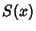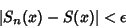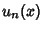## Uniform Convergence

A Seriesis uniformly convergent tofor a setof values ofif, for each, an Integercan be found such that(1)

forand all. To test for uniform convergence, use Abel's Uniform Convergence Test or the Weierstraß M-Test. If individual termsof a uniformly converging series are continuous, then
1. The series sum(2)

is continuous,

2. The series may be integrated term by term(3)

and

3. The series may be differentiated term by term(4)